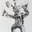Question-and-Answer Resource for the Building Energy Modeling Community
Get started with the Help page

# Suppose I have 100 variables calculated using Energyplus and I want to print the 100 in 10 CSV files, how may I achieve it ?Suppose I have 100 variables calculated using Energyplus and I want to print the 100 in 10 CSV files in such a way that each CSV file contains only 10 variables, how may I achieve it ?

edit retag close merge delete

Sort by » oldest newest most votedYou can use rvi input files to filter and order the variables from the eso output when ReadVarsESO produces the csv output. You can write a batch script to run ReadVarsESO multiple times on the same eso output, each with a different rvi input. There are many example rvi files in the EnergyPlus ExampleFiles folder, e.g. 1ZoneUncontrolled.rvi. See "Using ReadVarsESO" in the Input Output Reference for more information.

more

Python and pandas would do that quite easily. This code will output as many csv files as needed regardless of your actual number of variables, each file with 10 variables (except the latest which could have between 1 and 10). Each will be named like eplusout_0.csv, eplusout_1.csv, etc.

import pandas as pd
import numpy as np

# Chunck size
n = 10
for i in range(0, df.shape, n):
df_chunck = df.iloc[:, i:i+n]
df_chunck.to_csv('eplusout_{}.csv'.format(i))


Note: If you wanted, you do a bit better to properly parse the Date/Time CSV column as a valid DateTimeIndex.

I'll allow myself to go into a small rant here: the time conventions used by E+ as a MAJOR pain. The Date/Time index doesn't include the year, but more importantly it uses end of timestep conventions AND labels midnight as "24:00". Try to parse that in Python, you'll see.

In 
def dateparse(x):
return pd.datetime.strptime("2005/{}".format(x.strip()), '%Y/%m/%d %H:%M:%S')

df = pd.read_csv('eplusout.csv', index_col=0, parse_dates=True, date_parser=dateparse)

Out:
ValueError: time data '2005/01/01  24:00:00' does not match format '%Y/%m/%d %H:%M:%S'


This is because datetime logically expects 0-23 for hour.

In : import datetime
datetime.datetime(2005, 1, 1, 24, 0, 0)

Out: ValueError: hour must be in 0..23

more

1

The utility that is responsible for converting the ESO (which has dates and times that make sense internally to E+) into CSV is ReadVarsESO. Yes, it is in F90, but it is open source and we are happy to take contributions (even in other programming languages). The contribution policy is right here.

I would import the csv file into Excel and then separate whatever I need into different worksheets.

That should be quiet easy to do. You could write simple macro script to automate that.

You could also run simulation 10 times each time with different output list but that is inefficient.

more

Re Julien's rant above, regarding the poor E+ time conventions. Here is a snippet of python code that might be useful:

def read_eplusout(datafile, year=2018):

ep_datas={}
if os.path.exists(datafile):
with open(datafile,mode='r') as inf:

##Make sure that we read a variable that we want

##Creates a huge data structure
for i, lline in enumerate(inf):
tyy,thh=dd.split()
mm,dd=tyy.split('/')
hh,mmm,ss=thh.split(':')
if hh=='24':
else:
ddt=datetime.datetime(year,int(mm),int(dd),int(hh),int(mmm),int(ss))

continue

if dd=="":
dd=0.0

data_frame = pd.DataFrame(ep_datas)
data_frame = data_frame.set_index(pd.DatetimeIndex(ep_datas['Date/Time']))

return data_frame

more